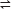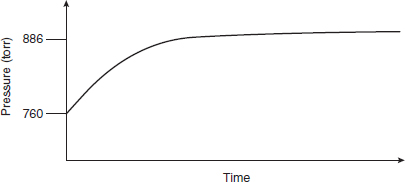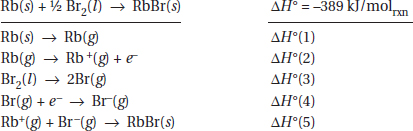# AP Chemistry Practice Test 25

### Test Information12 questions18 minutes

`Questions 1-5 refer to the following information.N2O4(g)2NO2(g)N2O4(g) decomposes into NO2(g) according to the equation above. A pure sample of N2O4(g) is placed into a rigid, evacuated, 0.500 L container. The initial pressure of the N2O4(g) is 760 atm. The temperature is held constant until the N2O4(g) reaches equilibrium with its decomposition products. The figure below shows how the pressure of the system changes while reaching equilibrium.`

1. Why does the pressure rise in this experiment?

2. The figure above gives us information about all the following except

3. By how much will the equilibrium pressure increase if this reaction goes to completion?

4. What can be said about the equilibrium constant, Kp, for this reaction?

5. Which diagram is an appropriate description of the system if more N2O(g) is rapidly injected, at time = 0, into the container after equilibrium is established?

`Questions 6-7 refer to the following information.This formation equation for the reaction synthesizing RbBr(s) can be separated into a series of steps.`

6. Which of the steps in the table above are endothermic?

7. If this reaction goes to completion, producing RbBr from the reactants, and if you use the overall chemical equation to estimate the entropy change for this process, which of the following statements is correct?

8. Using Hess's law, which of the following combinations will give the enthalpy for the following reaction?

Br2(l) + 2e- → 2Br-(g)

9. When 0.100 mol of Br2(l) is formed from RbBr in the reaction above, how much heat is released or absorbed?

10. If 1.0 g of rubidium and 1.0 g of bromine are reacted, what will be left in measurable amounts (more than 0.10 mg) in the reaction vessel?

11. Formation of a solution can often be visualized as a three-step process.

Step 1. Solvent molecules are separated from each other to make space for the solute.

Step 2. Solute molecules are separated so they fit into spaces in the solvent.

Step 3. Separated solute and solvent are brought together, filling in the spaces.

Which of the following statements about the energy involved in the above is correct?

12. Nickel (Z = 28, A = 59) has a first ionization energy of 737 kJ/mol and a boiling point of 2913 °C, while Pt (Z = 78, A = 195) has a first ionization energy of 870 kJ/mol and a boiling point of 3825 °C. Which of the following are the most reasonable values of ionization energy and boiling point for palladium?